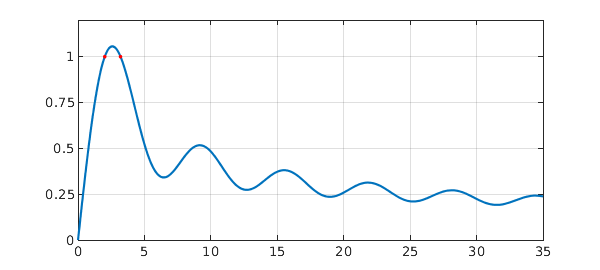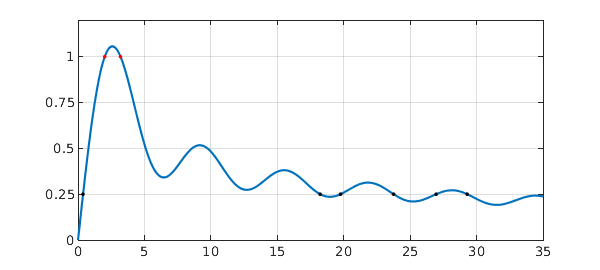This example shows how easy it is to solve one of the example problems from the Oxford MSc in Mathematical Modelling and Scientific Computing week 0 MATLAB 'Crash Course' using Chebfun. (And also how easy it is to make a Chebfun Example!).

Problem. For what values of $a$ does

$$I(a) = \int_{-1}^1 \sin(x) + \sin(a x^2) dx = 1 ?$$

Solution. Define the integrand as a function of $x$ and $a$.

F = @(x,a) sin(x) + sin(a*x.^2);

For a given $a$, we can compute the integral using Chebfun's sum command.

I = @(a) sum(chebfun(@(x) F(x,a)));

We compute a chebfun of this result, for $a$ ranging from $0$ to $100$.

Ia = chebfun(@(a) I(a),[0 100],'vectorize');

The 'vectorize' flag is used, since $I$ can not be evaluated with a vector.

We use Chebfun's roots command to find where $I(a)=1$.

r = roots(Ia-1)
r =
2.011698636650794
3.199526913460082


We plot this, to make sure it looks sensible.

LW = 'linewidth'; MS = 'markersize';
plot(Ia,LW,1.6), hold on, grid on
axis([0 35 0 1.2]), set(gca,'ytick',0:.25:1)
plot(r,Ia(r),'.r',MS,20);Since we have $I(a)$ as a chebfun, we can do other things, like find where $I(a) = 0.25$

r = roots(Ia-0.25)
plot(r,Ia(r),'.k',MS,20), hold off
r =
0.378866771015907
18.225950880000603
19.761174831761753
23.753831561562048
26.956276286229929
29.291546747613694or the value of $a$ which maximises $I(a)$

m = max(Ia)
m =
1.056688680049085


or the standard deviation of the gaps between the local minima for $a\in [0,100]$.

[y x] = min(Ia,'local');
f = std(diff(x(2:end-1)))
f =
0.008984009280336